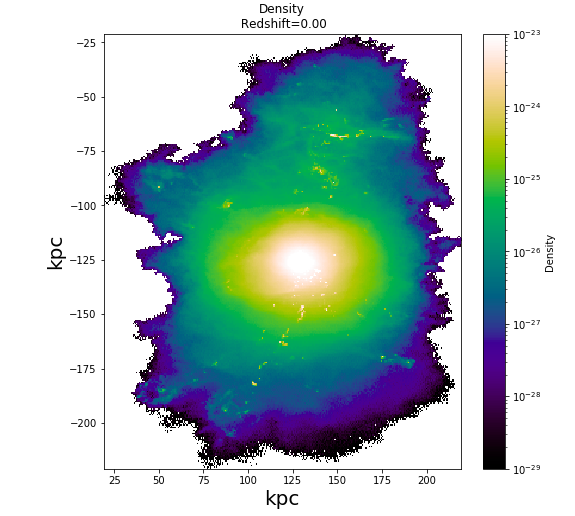### Calculating rotation measure for TNG 100-1 subhaloNikita Agarwal
• 16 Dec '21

Hi Dylan,

I have plotted a particular subhalo as a function of its density parameter (TNG 100-1 catalog).
Kindly request you to help me with the below queries:

`````` 1. How can I calculate the rotation measure value of the subhalo with the TNG 100-1 data available.

2. I have made my plot with the gas_pos[:,0] as x coordinates and gas_pos[:,2] as y coordinates. How can I find at what slice value of gas_pos[:,1] is the figure plotted?
``````

My code is as :

``````gas = il.snapshot.loadSubhalo(basePath,99 , 96500, 'gas', fields=None)

if ('Coordinates' in gas.keys() ):

a = 1 / (1 + header['Redshift'])
ckpc = kpc*a

gas = il.snapshot.loadSubhalo(basePath,99 , 96500, 'gas', fields=None)

gas_pos =(gas['Coordinates'])
sub_pos = subhalo['SubhaloPos']

gas_pos =(gas['Coordinates'])*ckpc/h  #kiloparsec
sub_pos =(subhalo['SubhaloPos'])*ckpc/h

x = (gas_pos[:,0] -  sub_pos)/kpc
y = (gas_pos[:,1] -  sub_pos)/kpc
z = (gas_pos[:,2] -  sub_pos)/kpc

xc = (np.max(x)+np.min(x))/2
yc = (np.max(y)+np.min(y))/2
zc = (np.max(z)+np.min(z))/2

rho = (gas['Density'])*((1e10*Msun/h)/(ckpc/h)**3)

make_plot1(rho,'cmr.rainforest',1e-29,1e-23,'Density','Density')

def make_plot1(param,color,lval,hval,title,label):
nPixels = [300,300]
extent = [xc-100,xc+100,zc-100,zc+100]
grid, _, _, _ = binned_statistic_2d(x,z, param, 'sum', bins=nPixels)

fig, ax = plt.subplots(figsize=[8,8])
im = ax.imshow(grid,extent = extent, cmap=color,interpolation='nearest', aspect = 'auto',origin = 'lower', norm = LogNorm(vmin = lval, vmax = hval))

plt.colorbar(im,label = label)
plt.xlabel('kpc',fontsize = 20)
plt.ylabel('kpc',fontsize = 20)
plt.show()
``````

My output is:Kind regards,
NikitaDylan Nelson
• 16 Dec '21

Dear Nikita,

(1) Do you mean the Faraday rotation measure? Then you will have to try to follow the mathematical definition of this quantity to calculate it. I'm afraid I don't have a simple solution to offer.

(2) If you create a 2D histogram of "x" versus "z" simulation coordinates versus each other, as you have done, and ignore the "y" coordinate, then you have effectively made a projection along this direction, including all cells (which you have loaded), regardless of their y-coordinate. Note that calculating a column density (summing masses, then dividing by the pixel area) may make more physical sense than calculating a sum of densities.Nikita Agarwal
• 19 Dec '21

Dear Dylan,

Will try this and reach out if I have further queries.

Kind regards,
NikitaNikita Agarwal
• 21 Dec '21

Dear Dylan,

Since in a 3D space, Density is a function of x,y,z is there any way to obtain 3D data separately for density for each of x,y, and z in TNG 100-1? Similarly, is there a way to obtain 3D data for Electron Abundance, Neutral Hydrogen Abundance, and for each component of Velocity?

Kind regards,
NikitaDylan Nelson
• 21 Dec '21

Dear Nikita,

You should think of the gas cells in the snapshot as a scattered point set, which provides samples of gas properties (including all those you mentioned, please see documentation) at x,y,z positions in space (given by the Coordinates dataset).

• Page 1 of 1